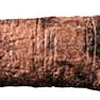#### You may also like### Where Can We Visit?

Charlie and Abi put a counter on 42. They wondered if they could visit all the other numbers on their 1-100 board, moving the counter using just these two operations: x2 and -5. What do you think?### Babylon Numbers

Can you make a hypothesis to explain these ancient numbers?### Ishango Bone

Can you decode the mysterious markings on this ancient bone tool?

# Arithmagons

##### Age 11 to 16Challenge Level

How does the sum of the numbers at the edges relate to the sum of the numbers at the vertices?

It might help to label the numbers at the vertices $A$, $B$ and $C$ and then express the edge numbers in terms of $A$, $B$ and $C$.